### Integers-Solutions Ex-6.2

CBSE Class –VI Mathematics
NCERT Solutions
Chapter 6
Integers (Ex. 6.2)

Question 1. Using the number line write the integer which is:
(a) 3 more than 5
(b) 5 more than –5
(c) 6 less than 2
(d) 3 less than –2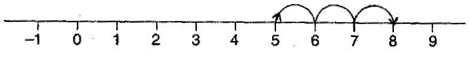(b) 0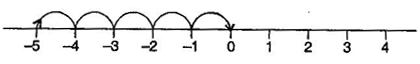(c) –4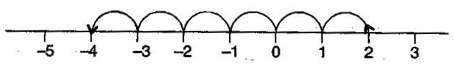(d) –5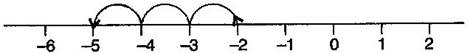Question 2. Use number line and add the following integers:
(a) 9 + (–6)
(b) 5 + (–11)
(c) (–1) + (–7)
(d) (–5) + 10
(e) (–1) + (–2) + (–3)
(f) (–2) + 8 + (–4)
Answer: (a) 9 + (–6) = 3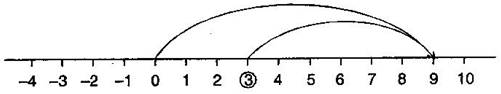(b) 5 + (–11) = –6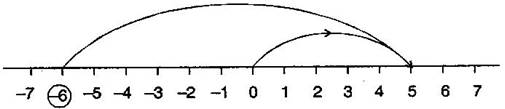(c) (–1) + (–7) = –8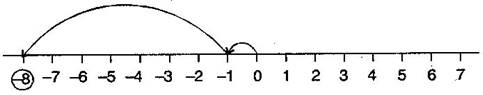(d) (–5) + 10 = 5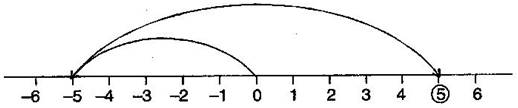(e) (–1) + (–2) + (–3) = –6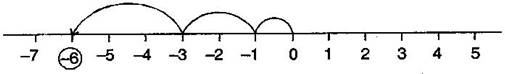(f) (–2) + 8 + (–4) = 2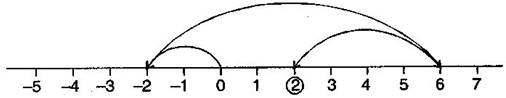Question 3. Add without using number line:
(a) 11 + (–7)
(b) (–13) + (+18)
(c) (–10) + (+19)
(d) (–250) + (+150)
(e) (–380) + (–270)
(f) (–217) + (–100)
Answer: (a) 11 + (–7) = 11 – 7 = 4
(b) (–13) + 18 = 5
(c) (–10) + (+19) = –10 + 19 = 9
(d) (–250) + (+150) = –250 + 150 = –100
(e) (–380) + (–270) = –380 – 270 = –650
(f) (–217) + (–100) = –217 -100 = –317
Question 4. Find the sum of:
(a) 137 and –354
(b) –52 and 52
(c) –312, 39 and 192
(d) –50, –200 and 300
Answer: (a) 137 + (–354) = 137 – 354 = –217
(b) (–52) + 52 = 0
(c) (–312) + 39 + 192 = –312 + 231 = –81
(d) (–50) + (–200) + 300 = –50 – 200 + 300 = –250 + 300 = 50
Question 5. Find the value of:
(a) (–7) + (–9) + 4 + 16
(b) 37 + (–2) + (–65) + (–8)
Answer: (a) (–7) + (–9) + 4 + 16
= –7 – 9 + 4 + 16
= –16 + 20
= 4
(b) 37 + (–2) + (–65) + (–8)
= 37 – 2 – 65 – 8
= 37 – 75
= –38